# 9 Interactive Manipulation

 9 Interactive Manipulation
The slider lets you pick values of n between 1 and 5 (in steps of 1):
Manipulate[Table[Orange, n], {n, 1, 5, 1}]

Here’s the whole sequence of possible results:
Table[Table[Orange, n], {n, 1, 5, 1}]

Here’s a similar interface for displaying a column of powers of a number:
Manipulate[Column[{n, n^2, n^3}], {n, 1, 10, 1}]

And here’s the list of possible results in this case:
Table[Column[{n, n^2, n^3}], {n, 1, 10, 1}]

Unlike Table, Manipulate isn’t limited to a fixed set of possible values. If you simply omit the step size in Manipulate, it’ll assume you want any possible value in the range, not limited to whole numbers.
Manipulate[Column[{n, n^2, n^3}], {n, 1, 10}]

It’s very common to have graphics that you want to adjust interactively.
A bar chart that changes as you move the slider:
Manipulate[BarChart[{1, a, 4, 2*a, 4, 3*a, 1}], {a, 0, 5}]

A pie chart of the same list:
Manipulate[PieChart[{1, a, 4, 2*a, 4, 3*a, 1}], {a, 0, 5}]

Manipulate lets you set up any number of controls. You just give the information for each variable in turn, one after another.
Build an interface to vary the number of sides, and the hue, of a polygon:
Manipulate[ Graphics[Style[RegularPolygon[n], Hue[h]]], {n, 5, 20, 1}, {h, 0, 1}]

There are many ways to specify controls for Manipulate. If you give a list of possible values, you’ll get a chooser or menu.
Build an interface that lets you choose between three colors:
Manipulate[ Graphics[ Style[RegularPolygon, color]], {color, {Red, Yellow, Blue}}]

Manipulate[ Style[value, color, size], {value, 1, 20, 1}, {color, {Black, Red, Purple}}, {size, Range[12, 96, 12]}]

 Manipulate[anything,{n,0,10,1}] manipulate anything with n varying in steps of 1 Manipulate[anything,{x,0,10}] manipulate anything with x varying continuously
9.1Make a Manipulate to show Range[n] with n varying from 0 to 100. »
Expected output:
 Out[]=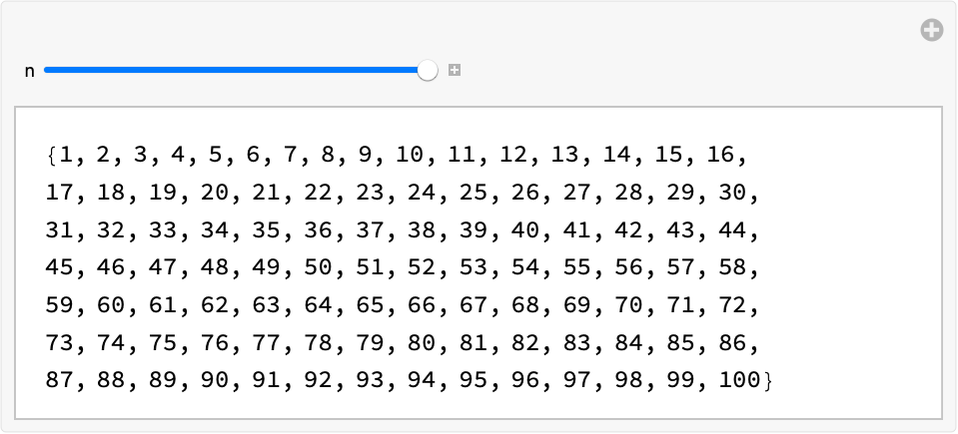Answer & check your solution
9.2Make a Manipulate to plot the whole numbers up to n, where n can range from 5 to 50. »
Expected output:
 Out[]=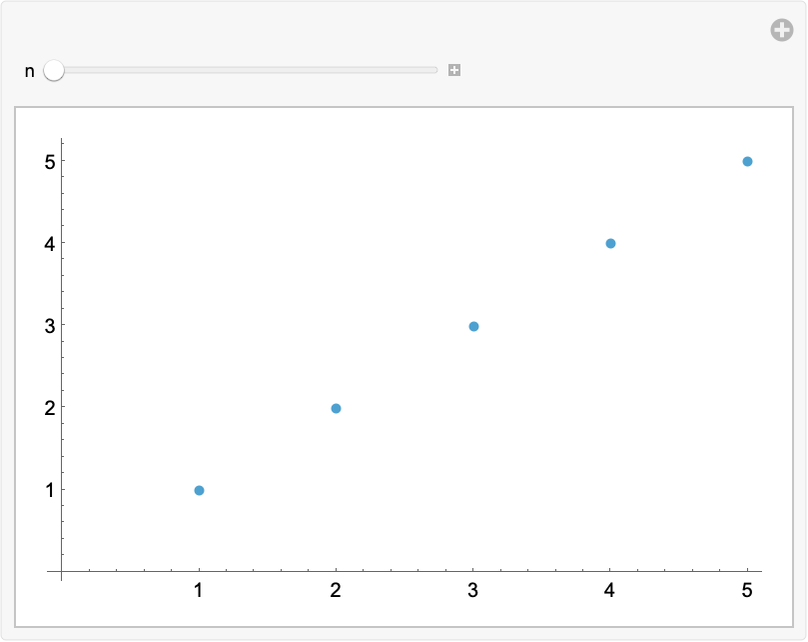Answer & check your solution
9.3Make a Manipulate to show a column of between 1 and 10 copies of x»
Expected output:
 Out[]=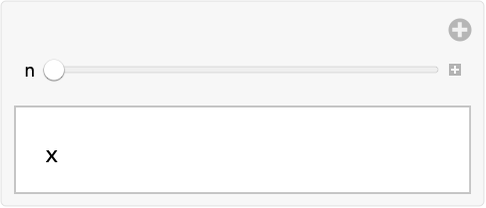Answer & check your solution
9.4Make a Manipulate to show a disk with a hue varying from 0 to 1. »
Expected output:
 Out[]=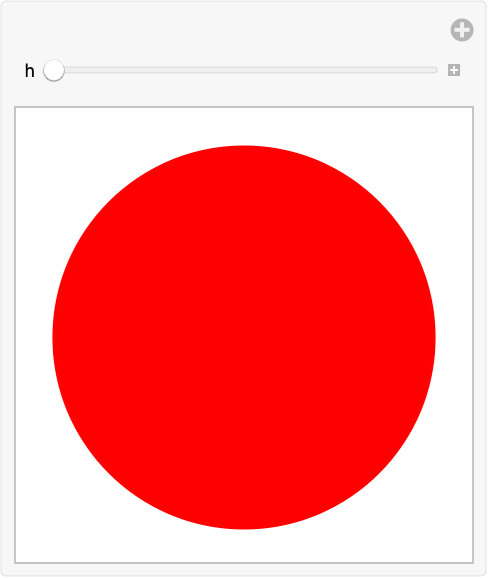Answer & check your solution
9.5Make a Manipulate to show a disk with red, green and blue color components varying from 0 to 1. »
Expected output:
 Out[]=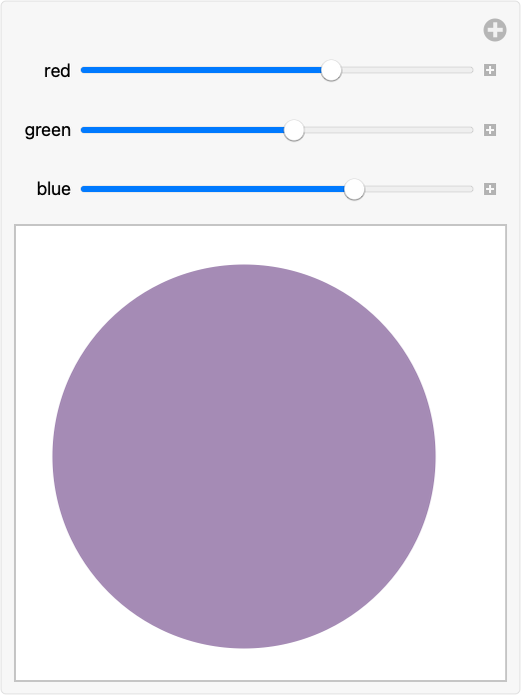Answer & check your solution
9.6Make a Manipulate to show digit sequences of 4-digit integers (between 1000 and 9999). »
Expected output:
 Out[]=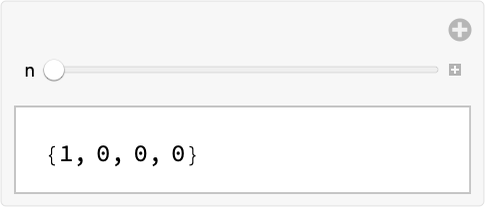Answer & check your solution
9.7Make a Manipulate to create a list of between 5 and 50 equally spaced hues. »
Expected output:
 Out[]=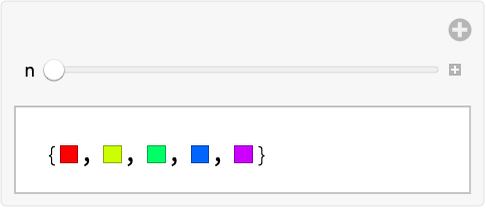Answer & check your solution
9.8Make a Manipulate that shows a list of a variable number of hexagons (between 1 and 10), and with variable hues. »
Expected output:
 Out[]=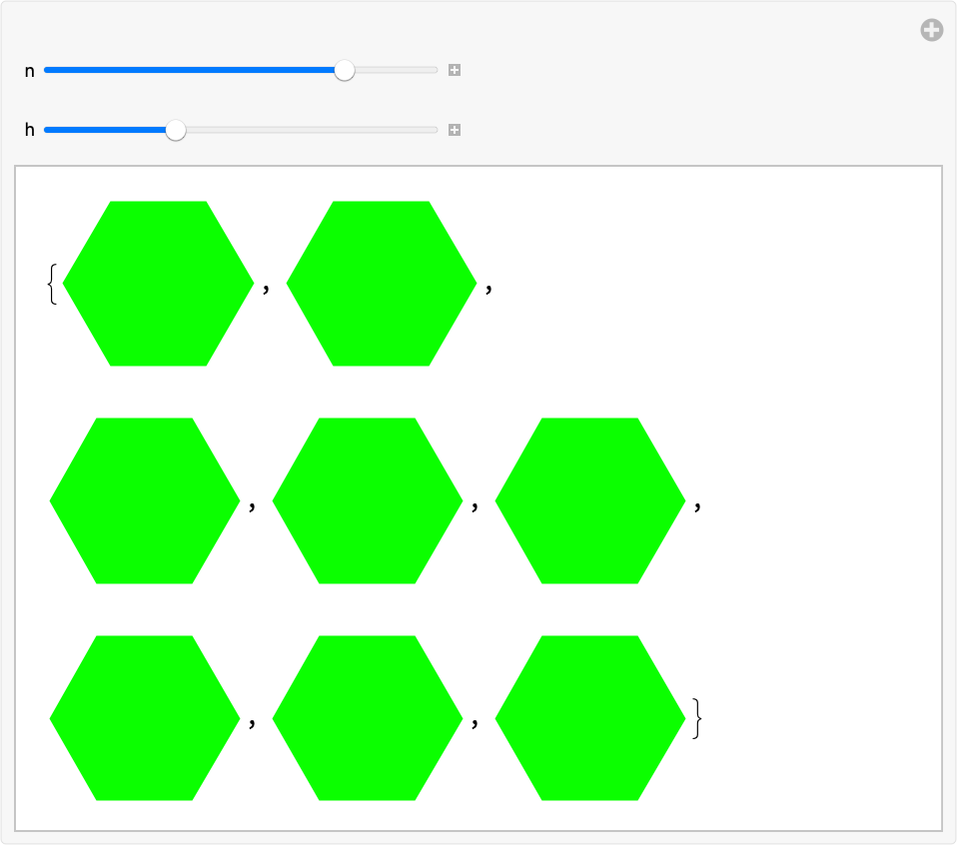Answer & check your solution
9.9Make a Manipulate that lets you show a regular polygon with between 5 and 20 sides, in red, yellow or blue. »
Expected output:
 Out[]=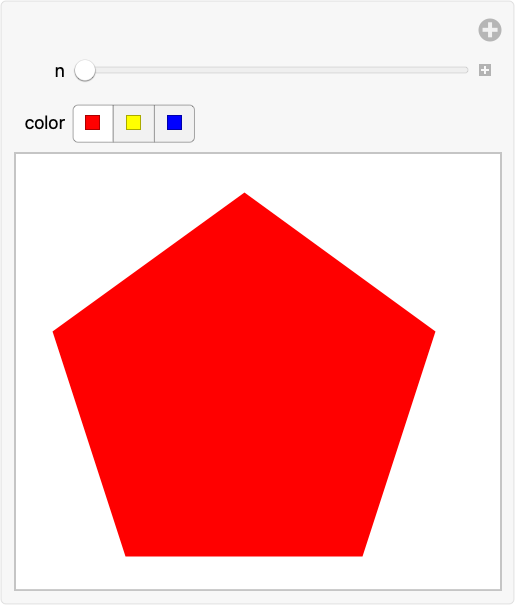Answer & check your solution
9.10Make a Manipulate that shows a pie chart with a number of equal segments varying from 1 to 10. »
Expected output:
 Out[]=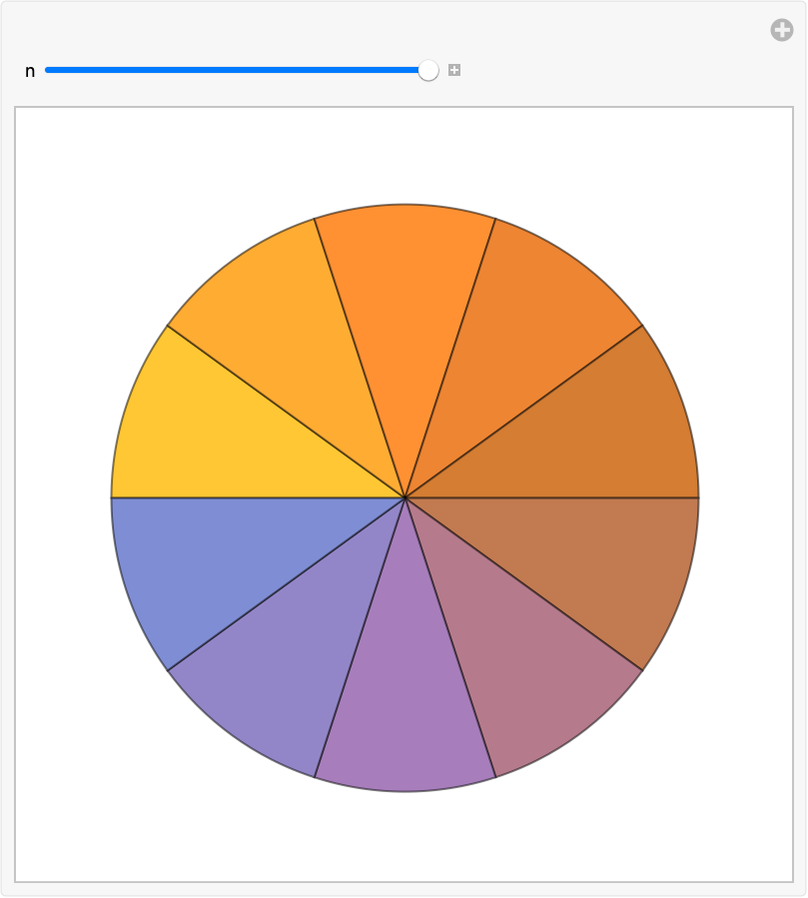Answer & check your solution
9.11Make a Manipulate that gives a bar chart of the 3 digits in integers from 100 to 999. »
Expected output:
 Out[]=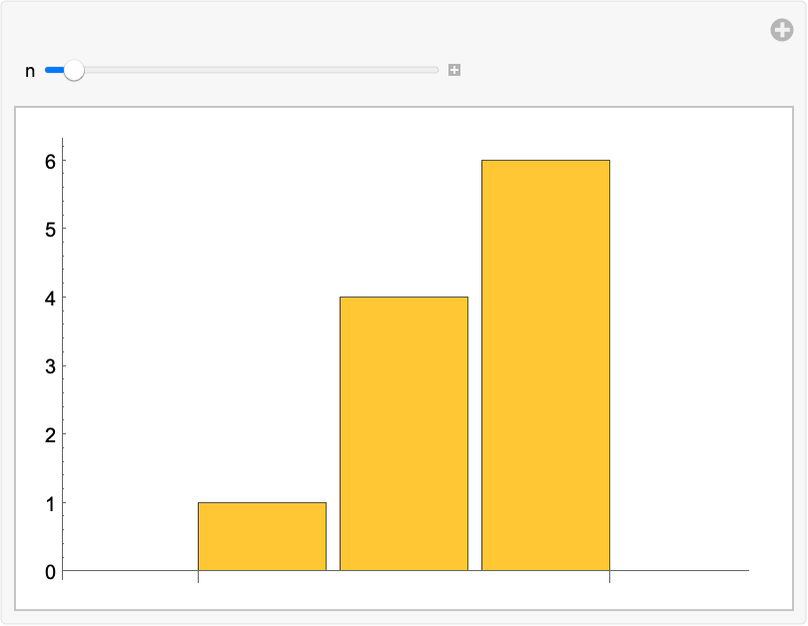Answer & check your solution
9.12Make a Manipulate that shows n random colors, where n can range from 1 to 50. »
Sample expected output:
 Out[]=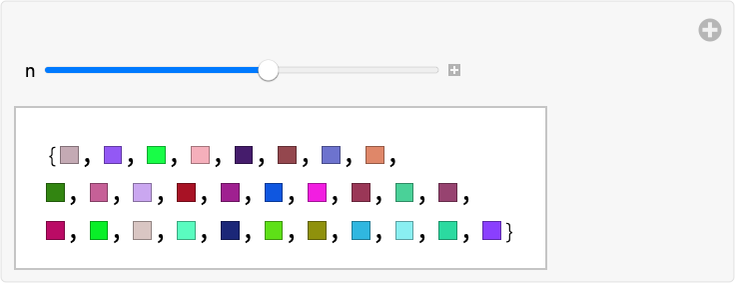Answer & check your solution
9.13Make a Manipulate to display a column of integer powers with bases from 1 to 25 and exponents from 1 to 10. »
Expected output:
 Out[]=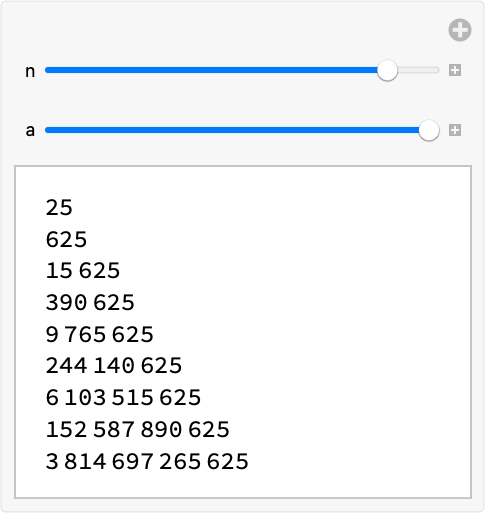Answer & check your solution
9.14Make a Manipulate of a number line of values of x^n for integer x from 1 to 10, with n varying from 0 to 5. »
Expected output:
 Out[]=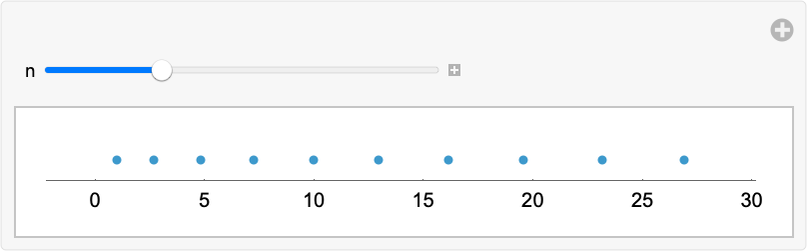Answer & check your solution
9.15Make a Manipulate to show a sphere that can vary in color from green to red. »
Expected output:
 Out[]=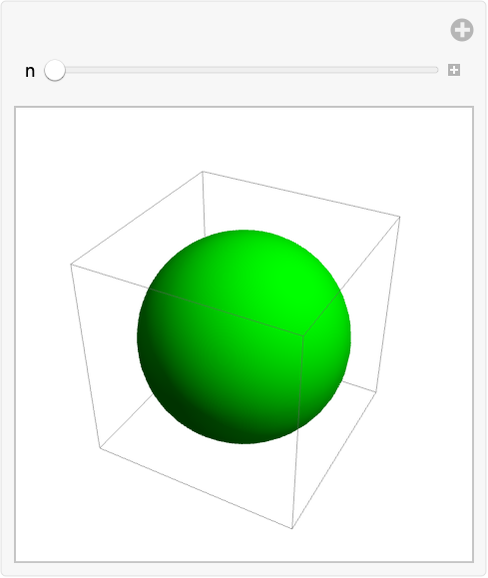Answer & check your solution
+9.1Make a Manipulate to plot numbers from 1 to 100 raised to powers that can vary between 1 and +1. »
Expected output:
 Out[]=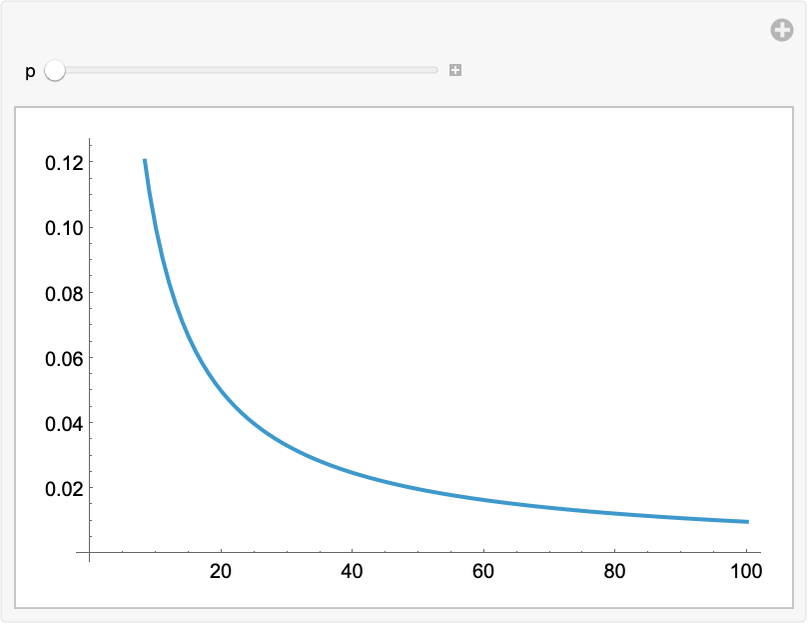Answer & check your solution
+9.2Make a Manipulate to display 1000 at sizes between 5 and 100. »
Expected output:
 Out[]=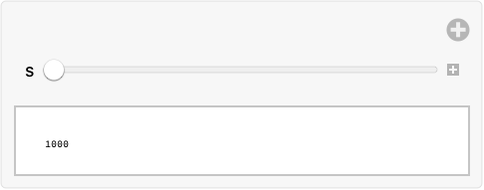Answer & check your solution
+9.3Make a Manipulate to show a bar chart with 4 bars, each with a height that can be between 0 and 10. »
Expected output:
 Out[]=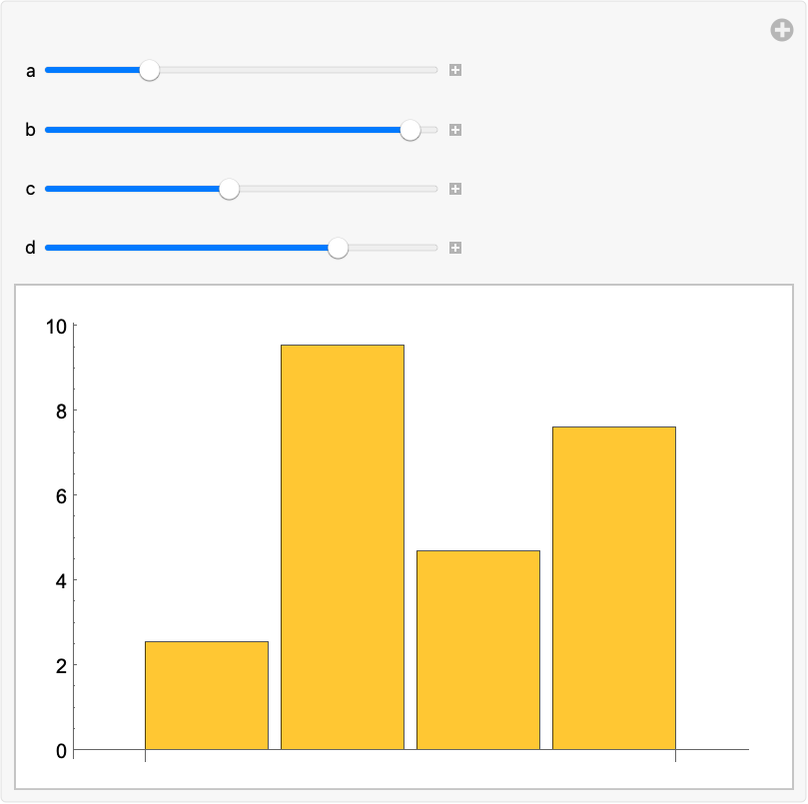Answer & check your solution
Does Manipulate work the same on web, mobile and desktop?
Ultimately yes. But it can be significantly slower on the web and on some mobile systems, because every time you move a slider it has to communicate over the internet with a server to find out what to do. On desktop and some mobile systems, everything is happening right there, inside your computer or other deviceso it’s very fast.
Can I make a standalone app out of a Manipulate?
Yes. To make a web app, for example, you just need to use CloudPublish. We’ll talk about this in Section 36.
Can I use random numbers in Manipulate?
Yes, but unless you “seed” them with SeedRandom, the random numbers will be different every time you move a slider.
• Manipulate supports pretty much all standard user interface control types (checkboxes, menus, input fields, color pickers, etc.).
• Sliders in Manipulate often provide a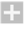button which opens out to additional controlsincluding animation, single stepping and numerical value display.
• Many controls are rendered differently on mouse and touch devices, and some controls only work on one or the other.
• If you’re running natively on your computer, devices like gamepads should immediately work with Manipulate. You can specify which controls should be hooked to what. gives information on all your controllers.
The Wolfram Demonstrations Project: more than 12,000 interactive Demonstrations created with Manipulate